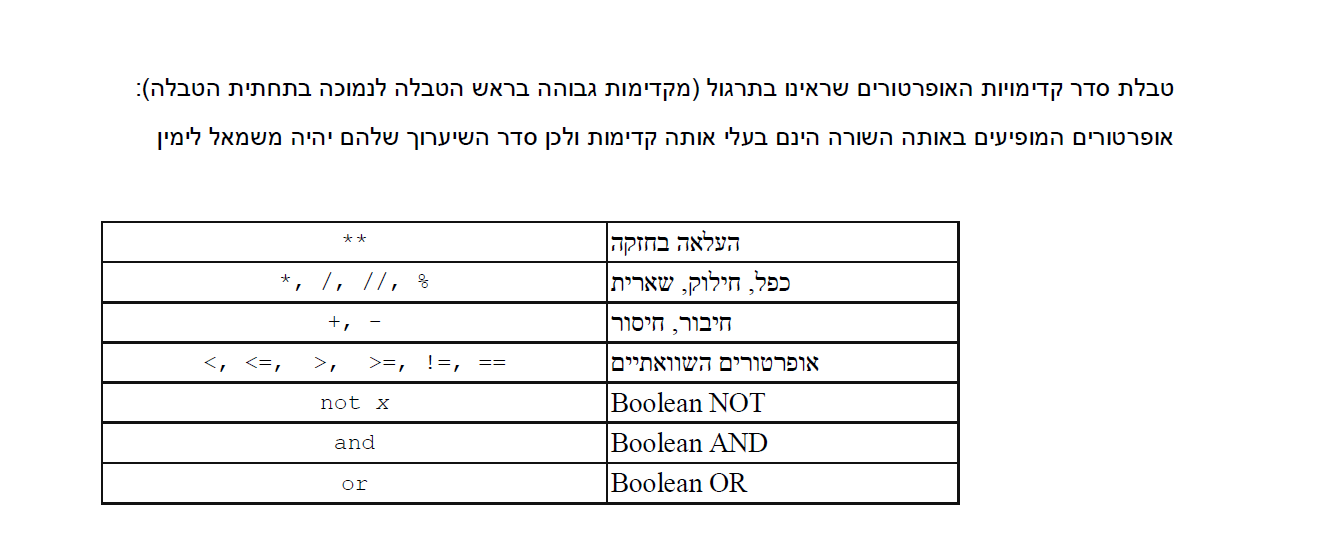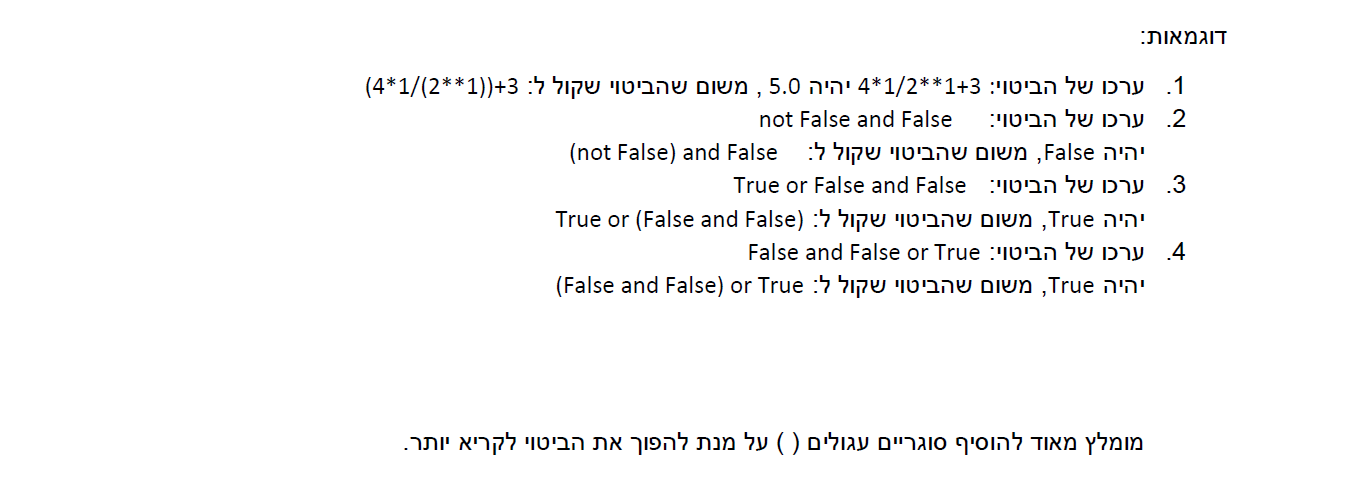# Recitation 1: Python basics¶

We discussed some basic types and operations, conversion functions, the function input and several structures that Python has to offer: if-else, while loops, and for loops. We also solved an excercise of counting the number of zeros in a number that is given as an input by the user.

Takeaways:

1. Try to "play" with types, operators, structures, and built-in functions in order to understand the basics of Python.
2. To tackle programming exercises, try to first imagine what you want the solution to do intuitively and then find ways to implement it.
3. Read the instructions for the submission of home assignments and submit your code as a .py file.
4. Test your code before you submit.

## IDLE¶

We saw a demonstration on how to use IDLE (needed for homework coding part). Don't forget to run the test() function that is already given in the skeleton file.

In order to open a .py file in Script mode, rightclick the file and select "Edit with IDLE".

### Working with notebooks¶

Read the following instructions if you are interested in working with Jupyter notebooks.

Code for printing several outputs in one cell (not part of the recitation):

In :
from IPython.core.interactiveshell import InteractiveShell
InteractiveShell.ast_node_interactivity = "all"


Types: int, float, string, bool

## Types and Variables¶

In :
x = 3
y = 3.14
name = "michal"
b = True
print(type(x))
print(type(y))
print(type(name))
print(type(b))
type(x)

<class 'int'>
<class 'float'>
<class 'str'>
<class 'bool'>

Out:
int

## Operators¶

In :
type("4")

Out:
str
In :
10 / 3

Out:
3.3333333333333335
In :
10 // 3

Out:
3
In :
10 // 2.0

Out:
5.0
In :
4 + 5.0

Out:
9.0
In :
2 ** 0.5

Out:
1.4142135623730951
In :
9 ** 0.5

Out:
3.0
In :
"4" + "5"

Out:
'45'
In :
"4" * 3

Out:
'444'
In :
"4" * "5"

---------------------------------------------------------------------------
TypeError                                 Traceback (most recent call last)
<ipython-input-13-ab0f866df984> in <module>
----> 1 "4" * "5"

TypeError: can't multiply sequence by non-int of type 'str'
In :
10 % 2

Out:
0
In :
2 % 10

Out:
2
In :
(-2) % 10

Out:
8
In :
3 < 4

Out:
True
In :
3 < 4 and 3 > 5

Out:
False

Task: find the rightmost digit in a given number, using the operators mentioned above:

In :
x = 345
# rightmost digit
x % 10
# second rightmost digit
(x // 10) % 10

Out:
5
Out:
4

Operator precedence:## Conversions¶

In :
int(3.14)

Out:
3
In :
int("3")

Out:
3
In :
float("3")

Out:
3.0
In :
float("3.14")

Out:
3.14
In :
str(3)

Out:
'3'
In :
str(3.14)

Out:
'3.14'

## If-else structure¶

In :
hungry = True
today = "Thursday"
tv_night =  "Thursday" #"Sunday"
last_episode_seen = 5

if hungry:
print("Better find something to eat...")
if today == "Friday":
print("Go to a restaurant")
else:
print("Cook something")
elif today == tv_night:
print("Watch episode number", last_episode_seen + 1, "of your favorite show")
elif today == "Thursday":
print("Go out for drinks!")
elif today == "Monday" or today == "Wednesday":
print("Workout")
elif today == "Friday" or today == "Tuesday" or today == "Saturday":
print("Go to sleep early :(")
else:
print("That's not a real day")

Better find something to eat...
Cook something


## Another If-elif-else example¶

In :
n = 100
if n % 2 == 0:
print("hi")
if n > 2:
print("bye")
else:
print("something")
print("end of if structure")

hi
bye
end of if structure

In :
n = 101
if n % 2 == 0:
print("hi")
if n > 2:
print("bye")
else:
print("something")
print("end of if structure")

bye
end of if structure

In :
n = 100
if n % 2 == 0:
print("hi")
elif n > 2:
print("bye")
else:
print("something")
print("end of if structure")

hi
end of if structure


## The function input()¶

In :
s = input("Please enter an integer: ")
print(s, type(s))

Please enter an integer: 3
3 <class 'str'>

In :
num = int(input("Please enter an integer: "))
print(num, type(num))

Please enter an integer: 3
3 <class 'int'>

In :
num = int(input("Please enter an integer: "))
print(num, type(num))

Please enter an integer: 3.2

---------------------------------------------------------------------------
ValueError                                Traceback (most recent call last)
<ipython-input-32-dedbc0dd1f23> in <module>
----> 1 num = int(input("Please enter an integer: "))
2 print(num, type(num))

ValueError: invalid literal for int() with base 10: '3.2'
In :
num = int(input("Please enter an integer: "))
print(num, type(num))

Please enter an integer: hi

---------------------------------------------------------------------------
ValueError                                Traceback (most recent call last)
<ipython-input-33-dedbc0dd1f23> in <module>
----> 1 num = int(input("Please enter an integer: "))
2 print(num, type(num))

ValueError: invalid literal for int() with base 10: 'hi'

## Introducing loops!¶

### While loop example: print all integers between 0 to 9¶

In :
i = 0
while i < 10:
print(i)
i = i + 1
print("out of loop, value of i is", i)

0
1
2
3
4
5
6
7
8
9
out of loop, value of i is 10


### Counting the number of zeros in a given integer (solution 1)¶

In :
print("This program will tell you how many 0's are in an integer")
orig_num = int(input("Please enter an integer: "))

num = orig_num
cnt = 0

while num > 0:
if num % 10 == 0:
cnt = cnt + 1 #cnt += 1
num = num // 10 #num //= 10

print(orig_num, "has", cnt, "0-s")

This program will tell you how many 0's are in an integer
1203 has 1 0-s


Are there any issues with the code above?

In :
print("This program will tell you how many 0's are in an integer")
orig_num = int(input("Please enter an integer: "))

num = orig_num
cnt = 0

while num > 0:
if num % 10 == 0:
cnt = cnt + 1 #cnt += 1
num = num // 10 #num //= 10

print(orig_num, "has", cnt, "0-s")

This program will tell you how many 0's are in an integer
0 has 0 0-s

In :
print("This program will tell you how many 0's are in an integer")
orig_num = int(input("Please enter an integer: "))

num = orig_num
cnt = 0

if num == 0:
cnt = 1

while num > 0:
if num%10 == 0:
cnt = cnt + 1 # cnt += 1
num = num // 10 #num //= 10

print (orig_num, "has", cnt , "0-s")

This program will tell you how many 0's are in an integer
0 has 1 0-s


### Understanding the code: tracking the values of the variables in this program¶

Supose that the user entered 1203. We will track the values of the variables using a table:

 orig_num num cnt Program output Before the loop 1203 1203 0 After iteration # 1 1203 120 0 After iteration # 2 1203 12 1 After iteration # 3 1203 1 1 After iteration # 4 1203 0 1 After the loop 1203 0 1 "1203 has 1 0-s"

### For loop example:¶

In :
for x in "michal":
print(x)
print(type(x))
print(x * 4)

m
<class 'str'>
mmmm
i
<class 'str'>
iiii
c
<class 'str'>
cccc
h
<class 'str'>
hhhh
a
<class 'str'>
aaaa
l
<class 'str'>
llll


### Counting the number of zeros in a given integer (solution 2)¶

In :
print("This program will tell you how many 0's are in an integer")
num_str = input("Please enter an integer: ")

cnt = 0

for digit in num_str:
if digit == "0":
cnt += 1

print (num_str, "has", cnt, "0-s")

This program will tell you how many 0's are in an integer
1203 has 1 0-s

In :
print("This program will tell you how many 0's are in an integer")
num_str = input("Please enter an integer: ")
cnt = 0

for digit in num_str:
if digit == "0":
cnt = cnt + 1

print(num_str, "has", cnt, "0-s")

This program will tell you how many 0's are in an integer
0 has 1 0-s


### Counting the number of zeros in a given integer (solution 3)¶

In :
num_str = input("Please enter an integer: ")
cnt = str.count(num_str, "0")
#cnt = num_str.count("0")
print (num_str, "has", cnt, "0's")

Please enter an integer: 1203
1203 has 1 0's

In [ ]: